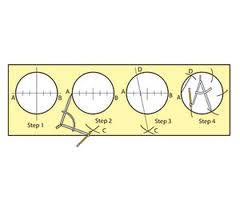## Divide a Circle into Five or Seven Equal Parts Assignment Help

Assignment Help: >> Circle and Curves - Divide a Circle into Five or Seven Equal Parts

To Divide a Circle into Five or Seven Equal Parts:

The component shown in diagram (a) is a threaded die. To sketch it, a circle has to be divided into five equal parts as depicted in diagram (b). First draw a horizontal line and mark on it point O. After that from point O like the center, describe a circle of the given diameter. From point A like the center and along with a radius equal to AO, sketch an arc to intersect at point n. From such point n, drop a perpendicular NC to the horizontal axis. From point C and along with a radius equal to C1, sketch an arc to intersect the horizontal axis at point m. Now from point 1 as the center and along with a radius the same to M1 draw an arc to intersect the circle at point 2. The latter or point 2 is the second desired point, point 1 being the first. The rest of the points dividing the circle into five (5) equal parts or points 3, 4 and 5 are found with setting the compass to the length 12 and marking off on the circle.The component shown in diagram (a) has seven equal-spaced holes. To divide a circle into seven equal parts, first draw a horizontal axis as in diagram 2.21(b). From point O like the center and along with the given radius, draw a circle. Sketch a vertical axis intersecting the circle at 1. From point A as the center and along with the radius OA, draw an arc to intersect the circle at point n. That from point n, drop a perpendicular to the horizontal axis to intersect it at point C. Set a length nC in the compass and from point 1, mark off this length on the circle to get the desired points 2, 3, 4, 5, 6 and 7. Join 1, 2, 3, 4, 5, 6 and 7 along with O to get seven equal parts.#### Assured A++ Grade

Get guaranteed satisfaction & time on delivery in every assignment order you paid with us! We ensure premium quality solution document along with free turntin report!

All rights reserved! Copyrights ©2019-2020 ExpertsMind IT Educational Pvt Ltd